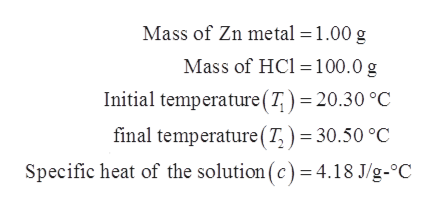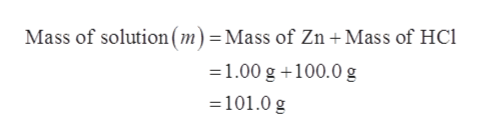# Zn metal reacts with hydrochloric acid according to the balanced equation Zn(s) + 2 HCl(aq) ĺZnCl2(aq) +H2(g) . When 1.00 g of Zn metal is completely reacted with 100.0 g of 0.500 M HCl(aq) in a constant-pressure calorimeter, the temperature of the solution was observed to rise from 20.30ºC to 30.50ºC. What is the change in enthalpy for the reaction (as written) per mole of Zn(s)? Use 4.18 J/g·ºC for the specific heat capacity of the solution. Assume no heat is lost to the surroundings.

Question
18 views

Zn metal reacts with hydrochloric acid according to the balanced equation Zn(s) + 2 HCl(aq) ĺZnCl2(aq) +H2(g) . When 1.00 g of Zn metal is completely reacted with 100.0 g of 0.500 M HCl(aq) in a constant-pressure calorimeter, the temperature of the solution was observed to rise from 20.30ºC to 30.50ºC. What is the change in enthalpy for the reaction (as written) per mole of Zn(s)? Use 4.18 J/g·ºC for the specific heat capacity of the solution. Assume no heat is lost to the surroundings.

check_circle

star
star
star
star
star
1 Rating
Step 1

Given data:help_outlineImage TranscriptioncloseMass of Zn metal =1.00 g Mass of HCl =100.0 g Initial temperature(T, ) = 20.30 °C final temperature(T, ) = 30.50 °C Specific heat of the solution (c) = 4.18 J/g-°C fullscreen
Step 2

When 1.00 g of Zn metal completely reacts with 100.0 g of HCl, it forms ZnCl2 and hydrogen gas. The corresponding reaction is shown below.

Step 3

Therefore, the mass of the solution is the sum of ...help_outlineImage TranscriptioncloseMass of solution(m) =Mass of Zn +Mass of HCl =1.00 g +100.0 g =101.0 g fullscreen

### Want to see the full answer?

See Solution

#### Want to see this answer and more?

Solutions are written by subject experts who are available 24/7. Questions are typically answered within 1 hour.*

See Solution
*Response times may vary by subject and question.
Tagged in

### Physical Chemistry